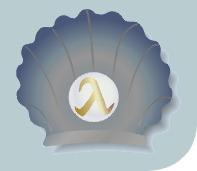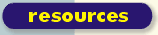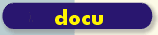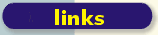scsh.net gmane.lisp.scheme.scsh 2003 [ Didn't bother to turn mime escapes of gb2312 codes into references to the corresponding chinese characters. Check out the ideogrammatic inscriptions in Zhao Wei's chinese article on the Scsh. ]```Message-ID: <3FA81505.7090904 at public1 ptt js cn> Date: Wed, 05 Nov 2003 05:07:17 +0800 From: ZHAO Wei To: scsh at zurich csail mit edu Subject: eyes-saver in scsh Hi, This is my first script in scsh. It is an eyes-saver which means if you are continuely working in front of a computer screen for longer than a specified time period, then a GTK+ 2 dialog will be pop-up and tell you that you must rest your eyes now, and after a very short time, when the dialog closes, the xscreensaver will be started. Any comments and suggestions are welcome! And I must thank the list for helping me sort out my questions on processes and threads which was posted earlier to this list. Thanks! The script depends on zenity and xscreensaver to work correctly. Both packages are available in Debian sid under the written names. -----------8<---------------- #! /usr/bin/scsh \ -o locks -o threads -e main -s !# ;;; Public Domain. ;;; ;;; ZHAO Wei (=D5=D4=CE=B5) ;;; 2003 Nov 04 ;;; ;;; QingQing (=C7=E4=C7=E4) The Eyes Tender ;;; Version 1.0 ;;; ;;; Depends on xscreensaver and zenity the GTK+ dialog displayer. ;;; Written for IBM developerWorks/China (define eyes-work-interval 3600) (define (display-line string) (display string) (newline)) (define (write-line thing) (write thing) (newline)) (define (make-locker val) (list (make-lock) val)) (define (locker-set! locker val) (let ((lock (car locker))) (obtain-lock lock) (set-cdr! locker val) (release-lock lock))) (define (locker-value locker) (let ((lock (car locker))) (obtain-lock lock) (let ((val (cdr locker))) (release-lock lock) val))) (define (work/check-delay work check delay) (let ((thread (lambda () (sleep (* delay 1000)) (if (check) (work))))) (spawn thread))) (define (watch-screensaver) (let ((blankq (list))) (let ((port (run/port (xscreensaver-command -watch))) (blankf (lambda () (for-each (lambda (f) (f)) blankq) (set! blankq (list)))) (unblankf (lambda () (let ((locker (make-locker #t))) (let ((uncheck (lambda () (locker-set! locker #f))) (check (lambda () (locker-value locker)))) (set! blankq (cons uncheck blankq)) (work/check-delay eyes-tender check eyes-work-interval)))))) (unblankf) (awk (read-line port) (line) () ((: bos "BLANK") (blankf)) ((: bos "UNBLANK") (unblankf)))))) (define (iconv str) (let ((ret (run/string (| (begin (display-line str)) (iconv -f gb2312 -t utf8))))) (substring ret 0 (- (string-length ret) 1)))) (define (eyes-tender) (let ((display-progress (lambda () (let lp ((progress 0)) (case progress ((1) (display-line (iconv "# =D1=DB=BE=A6=B8=C3=D0=DD=CF=A2=C0=B2= =A3=A1"))) ((50) (display-line (iconv "# =C2=ED=C9=CF=BE=CD=D2=AA=C6=F0=B6= =AF=C6=C1=C4=BB=B1=A3=BB=A4=B3=CC=D0=F2=C1=CB=A3=A1")))) (display-line progress) (if (< progress 100) (begin (sleep 60) (lp (+ 1 progress)))))))) (let ((ret (run (| (begin (display-progress)) (zenity --progress --width=3D300 --auto-close ,(string->symbol (string-append "--title=3D" (iconv "=C7=E4=C7=E4=B0=AE=BB=A4=C4=E3=B5=C4=D1=DB=BE=A6")))))) )) (if (=3D ret 0) (& (xscreensaver-command -activate)))))) (define (main ignore) (watch-screensaver)) ----------->8---------------- Thanks for reading so long! 8) --``` Up